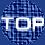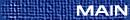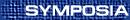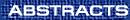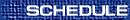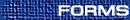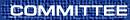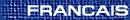Algebraic Groups / Groupes algébriques
(Carl Riehm, Organizer)

JAMES CARRELL, University of British Columbia
Singular loci of subvarieties of G/B stable under a maximal torus

Let G be a semi-simple algebraic group over an algebraically closed field of characteristic zero, and T Ì B Ì G a maximal torus and Borel subgroup respectively. We will state a general result about the set of smooth points of a closed subvariety Y Ì G/B stable under T which allows us to characterize the smooth points of a Schubert variety X in G/B. In particular, a Schubert variety X is nonsingular iff its reduced tangent cones are all linear. The reason this result is nontrivial is that the reduced tangent cone of an X at a point x almost never spans the tangent space to X at x. A corollary of this is a well known unpublished result of Dale Peterson that if G is simply laced, then every rationally smooth Schubert variety is in fact already smooth. (Joint work with Jochen Kuttler).

DRAGOMIR Z. DJOKOVI\'C, Waterloo, Ontario
Closure ordering of adjoint nilpotent orbits in simple real lie algebras

There are only finitely many adjoint nilpotent orbits O in a simple real Lie algebra g0. The closure of O is the union of O and some orbits of smaller dimension. I shall discuss the problem of deciding which orbits are contained in the closure of O. An approach to this problem via the Kostant-Sekiguchi correspondence will be described. Surprisingly, this problem is still open when g0 = so(p,q). Some partial results for this case were obtained in a joint work with J. Sekiguchi and N. Lemire. I shall also present the results, obtained so far, in the case when g0 is a noncompact real form of a simple exceptional complex Lie algebra.

BRUCE GILLIGAN, University of Regina, Regina, Saskatchewan
On globalization of holomorphic actions

Suppose P ® X is a holomorphic principal bundle and a Lie group G acts as a group of holomorphic automorphisms on the bundle P® X. We will show that if there exists a holomorphic action of the complexification G\mathbb C of G on X, then this lifts to a holomorphic action of G\mathbb C on the bundle P ®X. Applications of this will also be presented.

ANDY R. MAGID, University of Oklahoma, Oklahoma, USA
Pro-algebraic hulls of discrete groups: examples and questions

The pro-algebraic hull A(G) of a discrete group G is the inverse limit of the Zariski closures of all finite dimensional complex representations of G. We consider only finitely generated G whose representations separate the elements of G (in the finitely generated case, this is the same as residually finite). Examples are presented where the (pro)unipotent radical of A(G) is infinite dimensional and the reductive quotient finite dimensional, and where the prounipotent radical is trivial but A(G) is infinite dimensional and the occurrence of simple factors in A(G) of given dimension is bounded. (Joint work with H. Bass, A. Lubotzky, S. Mozes)

ARTURO PIANZOLA, University of Alberta, Edmonton, Alberta
Line bundles and conjugacy theorems

We will look at how the triviality of line bundles on Spec(R) is related to the conjugacy of Cartan subalgebras of g ÄR.

LEX E. RENNER, University of Western Ontario
An explicit cell decomposition of the canonical compactification of a semisimple algebraic group

By work of De Concini, Procesi and Springer, there exists a cell decomposition of X = [`(G)]:

 X = \bigsqcupa Î W ×W Ca
where W is the Weyl group and Ca @ Kma for some ma ³ 0. Each Ca is B×B-invariant. Furthermore, they calculate each ma, thereby yielding a formula for the Betti numbers of X.

We take a more direct approach. First we identify the set of B×B-orbits combinatorically in terms of (W, f, D), and then we define each Ca explicitly as a union of B×B-orbits. Our approach also determines a cell decomposition of each G×G-orbit closure XJ Í X, J Í D.

CARL RIEHM, McMaster University
Orthogonal representations of finite groups

If G ® GL(V) is a representation of the finite group G on the vector space V over a local field, we will describe the symmetric bilinear forms on V which are G-invariant, and count the number of orthogonally equivalent orthogonal representations for each of these invariant forms. Partial results for global fields will also be given.

JOHN SCHERK, Toronto

DAVID WEHLAU, Royal Military College of Canada and Queen's University
Using ladders to compute invariants of p-groups

Suppose that V is a finite dimensional vector space over the field k and that G is a finite subgroup of GL(V). There is an induced action of G on the ring of regular functions k[V]. The ring of invariants, k[V]G, is the finitely generated subring consisting of those functions fixed by the action of G. If the order of G is a unit in k, then k[V]G is reasonably well understood and there are fairly effective algorithms for constructing a minimal generating set. This is not the case if the characteristic of k divides the order of G.

Then there exists a normal subgroup, N, with G/N isomorphic to the cyclic group of order p, Z/p. The ring of invariants is given by

 k[V]G = (k[V]N)G/N = (k[V]N)Z/p.
Therefore it is possible, in principle, to construct a generating set inductively. In other words, to climb the ladder from k[V] to k[V]G. Each step up the ladder requires the computation of the Z/p-invariants of a finitely generated graded algebra. Computing Z/p-invariants can be difficult and this approach is not practical for an arbitrary p-group. There are, however, cases were the ladder approach works well.

I intend to discuss the elements required to make the ladder approach work. This involves some interesting group cohomology calculations. I will also describe a family of representations for which the ladder approach has proven to be more effective than any other currently known method. (Joint work with Dr R. James Shank (Institute of Mathematics & Statistics, University of Kent at Canterbury).